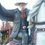# Some thoughts about the sums

On this note, I said about some patterns, even though the Faulhaber's formula makes this somewhat useless, I found some patters, first of all, as someone commented:

$\sum^n_{i=1}i^a\approx\frac{n^{a+1}}{a+1}$

I will prove the following statement:

"The highest order term of the polynomial that represents $\sum^n_{i=1}i^a$ is $\frac{n^{a+1}}{a+1}$"

I will use a strong induction to prove this, starting with the base case of $a=0$, we have:

$\displaystyle \sum^n_{i=1}i^a=n$ So the highest term is $n=\frac{n^{a+1}}{a+1}$

We will assume that this will work for $a=0,1,2,\ldots,k$, then for $a=k+1$, we have:

$\displaystyle \sum^n_{j=1}j^{k+1}=\frac{\sum^{k+2}_{i=1}\binom{k+2}{i}n^i-\sum^k_{i=0}\left (\binom{k+2}{i}\sum^n_{j=1}j^i \right )}{k+2}$

The highest term of this will be $\frac{n^{k+2}}{k+2}$, that is obtained when, on the first sum, the $i=k+2$, so $\binom{k+2}{i}n^i=\binom{k+2}{k+2}n^{k+2}=n^{k+2}$, no other term has this order on the numerator, because the highest one on the other sum is $\frac{n^{k+1}}{k+1}$, whose order is not the same, the term becomes $\frac{n^{k+2}}{k+2}$ when I consider the denominator;

The other pattern I saw is that the second term of the sum is $\frac{n^a}{2}$

I will have to use the Faulhaber's formula:

$\sum^n_{i=1}i^a=\frac{1}{a+1}\sum^a_{i=0}\binom{a+1}{i}B^+_in^{a+1-i}$

The term of $a$ degree appears when i=1 on the right sum, so it is:

$\frac{1}{a+1}\binom{a+1}{1}B^+_1n^a=B^+_1n^a$

Since $B^+_1=\frac{1}{2}$ (I am using this definition), the term is:

$\frac{1}{2}n^a=\frac{n^a}{2}$Note by Matheus Jahnke
4 years ago

This discussion board is a place to discuss our Daily Challenges and the math and science related to those challenges. Explanations are more than just a solution — they should explain the steps and thinking strategies that you used to obtain the solution. Comments should further the discussion of math and science.

When posting on Brilliant:

• Use the emojis to react to an explanation, whether you're congratulating a job well done , or just really confused .
• Ask specific questions about the challenge or the steps in somebody's explanation. Well-posed questions can add a lot to the discussion, but posting "I don't understand!" doesn't help anyone.
• Try to contribute something new to the discussion, whether it is an extension, generalization or other idea related to the challenge.

MarkdownAppears as
*italics* or _italics_ italics
**bold** or __bold__ bold
- bulleted- list
• bulleted
• list
1. numbered2. list
1. numbered
2. list
Note: you must add a full line of space before and after lists for them to show up correctly
paragraph 1paragraph 2

paragraph 1

paragraph 2

[example link](https://brilliant.org)example link
> This is a quote
This is a quote
    # I indented these lines
# 4 spaces, and now they show
# up as a code block.

print "hello world"
# I indented these lines
# 4 spaces, and now they show
# up as a code block.

print "hello world"
MathAppears as
Remember to wrap math in $$ ... $$ or $ ... $ to ensure proper formatting.
2 \times 3 $2 \times 3$
2^{34} $2^{34}$
a_{i-1} $a_{i-1}$
\frac{2}{3} $\frac{2}{3}$
\sqrt{2} $\sqrt{2}$
\sum_{i=1}^3 $\sum_{i=1}^3$
\sin \theta $\sin \theta$
\boxed{123} $\boxed{123}$

Sort by:

These are great thoughts! Mathematicians look for patterns in the data, and then try to prove that the pattern exists, as you have done above.

Staff - 4 years ago